# tfridge

Time-frequency ridges

## Syntax

``fridge = tfridge(tfm,f)``
``````[fridge,iridge] = tfridge(tfm,f)``````
``````[fridge,iridge,lridge] = tfridge(tfm,f)``````
``[___] = tfridge(tfm,f,penalty)``
``[___] = tfridge(___,'NumRidges',nr)``
``[___] = tfridge(___,'NumRidges',nr,'NumFrequencyBins',nbins)``

## Description

````fridge = tfridge(tfm,f)` extracts the maximum-energy time-frequency ridge from the time-frequency matrix, `tfm`, and the frequency vector, `f`, and outputs the time-dependent frequency, `fridge`.```
``````[fridge,iridge] = tfridge(tfm,f)``` also returns the row-index vector corresponding to the maximum-energy ridge.```

example

``````[fridge,iridge,lridge] = tfridge(tfm,f)``` also returns the linear indices, `lridge`, such that `tfm(lridge)` are the values of `tfm` along the maximum-energy ridge.```

example

````[___] = tfridge(tfm,f,penalty)` penalizes changes in frequency by scaling the squared distance between frequency bins by `penalty`.```
````[___] = tfridge(___,'NumRidges',nr)` extracts the `nr` time-frequency ridges with the highest energy. This syntax accepts any combination of input arguments from previous syntaxes.```

example

````[___] = tfridge(___,'NumRidges',nr,'NumFrequencyBins',nbins)` specifies the number of frequency bins around a ridge that are removed from `tfm` when extracting multiple ridges.```

## Examples

collapse all

Create a matrix that resembles a time-frequency matrix with a sharp ridge. Visualize the matrix in three dimensions.

```t = 0:0.05:10; f = 0:0.2:8; rv = 1; [F,T] = ndgrid(f,t); S = zeros(size(T)); S(abs((F-4)-cos((T-6).^2))<0.1) = rv; mesh(T,F,S) view(-30,60)```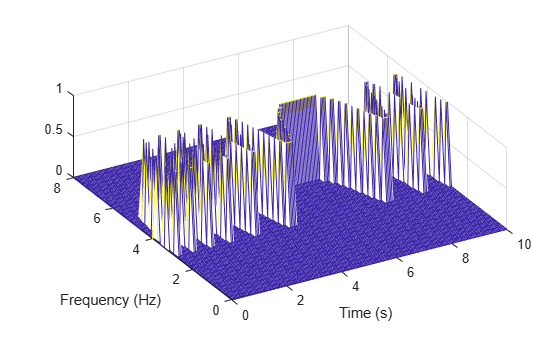Add noise to the matrix and redisplay the plot.

```S = S+rand(size(S))/10; mesh(T,F,S) view(-30,60) xlabel('Time') ylabel('Frequency')```Extract the ridge and plot the result.

```[fridge,~,lridge] = tfridge(S,f); rvals = S(lridge); hold on plot3(t,fridge,rvals,'k','linewidth',4) hold off```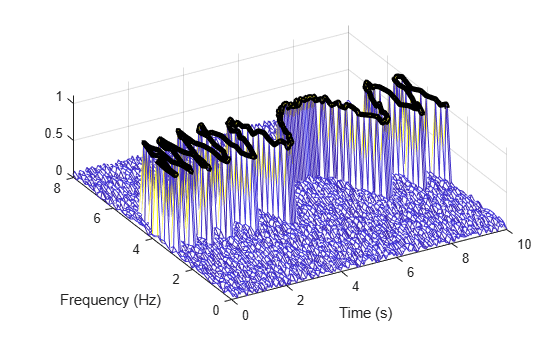Generate a signal that is sampled at 3 kHz for one second. The signal consists of two tones and a quadratic chirp.

• The first tone has a frequency of 1000 Hz and unit amplitude.

• The second tone has a frequency of 1200 Hz and unit amplitude.

• The chirp has an initial frequency of 500 Hz and reaches 750 Hz at the end of the sampling. It has an amplitude of six.

```fs = 3000; t = 0:1/fs:1-1/fs; x1 = 6*chirp(t,fs/6,t(end),fs/4,'quadratic'); x2 = sin(2*pi*fs/3*t); x3 = sin(2*pi*fs/2.5*t); x = x1+x2+x3;```

Compute and display the Fourier synchrosqueezed transform of the signal.

```[sst,f] = fsst(x,fs); mx = max(abs(sst(:)))*ones(size(t)); mesh(t,f,abs(sst)) view(2)```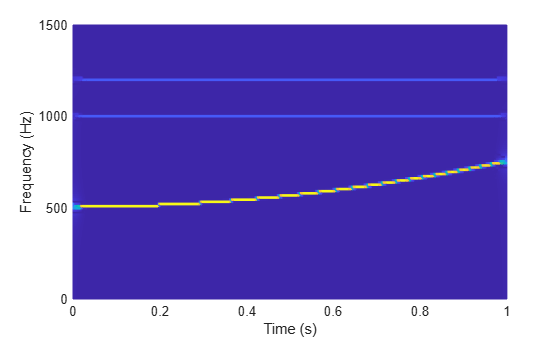Extract and plot the two highest-energy signal components. Set no penalty for changing frequency.

```penval = 0; fridge = tfridge(sst,f,penval,'NumRidges',2); hold on plot3(t,fridge,mx,'w','linewidth',5) hold off```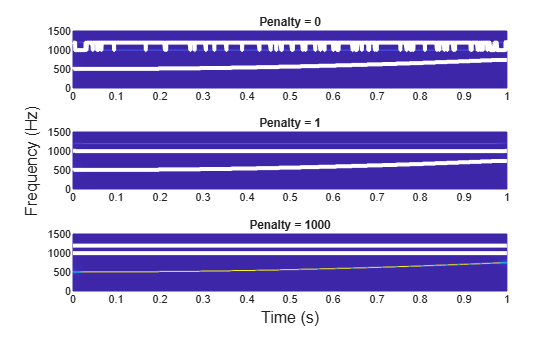The two tones have the same amplitude, and the algorithm jumps between them. Set the penalty for changing frequency to 1.

```penval = 1; fridge = tfridge(sst,f,penval,'NumRidges',2); mesh(t,f,abs(sst)) view(2) xlabel('Time (s)') ylabel('Frequency (Hz)') hold on plot3(t,fridge,mx,'w','linewidth',5) hold off```Set the penalty to a high value for comparison. The chirp is penalized because its frequency is not constant.

```penval = 1000; fridge = tfridge(sst,f,penval,'NumRidges',2); mesh(t,f,abs(sst)) view(2) xlabel('Time (s)') ylabel('Frequency (Hz)') hold on plot3(t,fridge,mx,'w','linewidth',5) hold off```Generate a signal composed of two quadratic chirps. The signal is sampled at 1 kHz for 3 seconds. The chirps are such that the instantaneous frequency is symmetric about the halfway point of the sampling interval. One chirp is concave and the other chirp is convex. The concave chirp has twice the amplitude of the convex chirp.

```fs = 1e3; t = 0:1/fs:3; x = chirp(t-1.5,100,1.1,200,'quadratic',[],'convex'); y = 2*chirp(t-1.5,300,1.1,400,'quadratic',[],'concave'); % To hear, type soundsc(x+y,fs)```

Compute and display the Fourier synchrosqueezed transform of the signal.

```sig = x+y; [sst,f,t] = fsst(sig,fs); fsst(sig,fs,'yaxis')```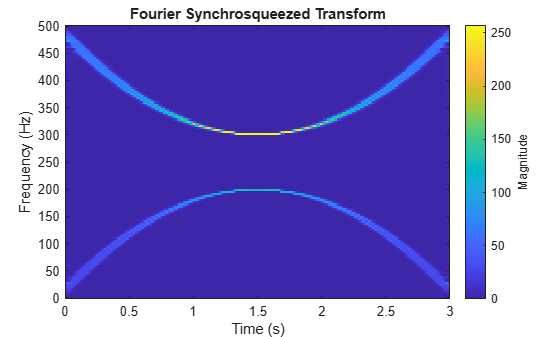Extract the two time-frequency ridges that have the highest energy. Specify a penalty of 1 for changes in frequency. Remove 1 frequency bin around the ridge with the highest energy before extracting the second ridge. Plot the ridges.

```nfb = 1; [fr,ir] = tfridge(sst,f,1,'NumRidges',2,'NumFrequencyBins',nfb); plot(t,fr)```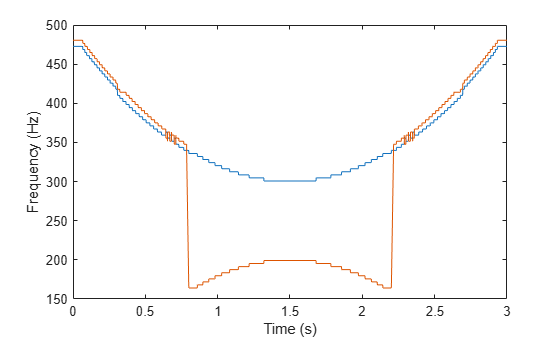One bin is insufficient: The function finds a second ridge that is partly on the slope of the first. Increase to 50 the number of bins to remove and repeat the calculation.

```nfb = 50; [fr,ir] = tfridge(sst,f,1,'NumRidges',2,'NumFrequencyBins',nfb); plot(t,fr)```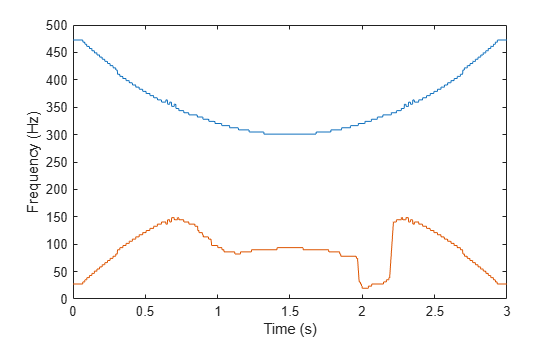Removing too many bins distorts lower-energy ridges. Decrease the number to 15 and repeat the calculation.

```nfb = 15; [fr,ir] = tfridge(sst,f,1,'NumRidges',2,'NumFrequencyBins',nfb); plot(t,fr)```Invert the transform corresponding to the two ridges. Add the ridges to reconstruct the signal. Plot the difference between the reconstructed signal and the chirps.

```itr = ifsst(sst,[],ir,'NumFrequencyBins',nfb); xrec = sum(itr'); plot(t,xrec-(x+y)) ylim([-.1 .1])```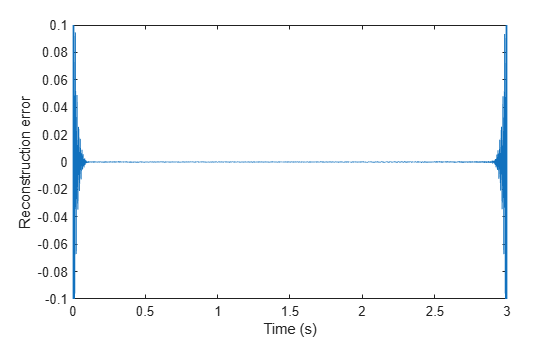`% To hear, type soundsc(xrec,fs)`

The agreement is good most of the time but deteriorates at the ends, where frequencies change most rapidly.

## Input Arguments

collapse all

Time-frequency matrix, specified as a matrix.

Example: `fsst(cos(pi/4*(0:159)))` specifies the synchrosqueezed transform of a sinusoid.

Data Types: `single` | `double`
Complex Number Support: Yes

Sampling frequencies, specified as a vector. The length of `f` must equal the number of rows in `tfm`.

Data Types: `single` | `double`

Penalty for changing frequency, specified as a nonnegative real scalar.

Data Types: `single` | `double`

Number of time-frequency ridges to extract, specified as the comma-separated pair consisting of `'NumRidges'` and a positive integer scalar. You can specify this name-value pair anywhere after `tfm` in the input argument list.

When `nr` is greater than 1, `tfridge`:

1. Extracts the time-frequency ridge with the highest energy

2. Removes from `tfm` the energy contained in the ridge it extracted and in the `nbins` adjacent frequency bins on either side of the ridge

3. Extracts the highest-energy ridge in the modified `tfm`

4. Iterates until it has extracted `nr` ridges

Data Types: `single` | `double`

Number of bins to remove when extracting multiple ridges, specified as the comma-separated pair consisting of `'NumFrequencyBins'` and a positive integer scalar. `nbins` must be smaller than 1/4 of the sampling frequencies. Indices close to the frequency edges that have fewer than `nbins` bins on one side are reconstructed using a smaller number of bins.

Data Types: `single` | `double`

## Output Arguments

collapse all

Time-frequency ridges, returned as a matrix with `nr` columns. The number of rows in `fridge` equals the number of columns in `tfm`. The first column contains the frequencies that correspond to the highest-energy ridge. Subsequent columns contain the frequencies of the other ridges in decreasing order of energy.

Ridge row indices, returned as a matrix with `nr` columns. The number of rows in `iridge` equals the number of columns in `tfm`. The first column contains the indices that correspond to the highest-energy ridge. Subsequent columns contain the indices of the other ridges in decreasing order of energy.

Ridge linear indices, returned as a matrix with `nr` columns. `lridge` is defined so that `tfm(lridge)` is the amplitude of `tfm` along the ridges. The number of rows in `lridge` equals the number of columns in `tfm`. The first column contains the indices that correspond to the highest-energy ridge. Subsequent columns contain the indices of the other ridges in decreasing order of energy.

Example: `lridge` is equivalent to `sub2ind(size(tfm),iridge,repmat((1:size(tfm,2))',1,nr))`.

## Algorithms

The function uses a penalized forward-backward greedy algorithm to extract the maximum-energy ridges from a time-frequency matrix. The algorithm finds the maximum time-frequency ridge by minimizing –ln A at each time point, where A is the absolute value of the matrix. Minimizing –ln A is equivalent to maximizing the value of A. The algorithm optionally constrains jumps in frequency with a penalty that is proportional to the distance between frequency bins.

The following example illustrates the time-frequency ridge algorithm using a penalty that is two times the distance between frequency bins. Specifically, the distance between the elements `(j,k)` and `(m,n)` is defined as `(j-m)2`. The time-frequency matrix has three frequency bins and three time steps. The matrix columns correspond to time steps, and the matrix rows correspond to frequency bins. The values in the second row represent a sine wave.

1. Suppose you have the matrix:

```1 4 4 2 2 2 5 5 4```

2. Update the value for the (1,2) element as follows.

1. Leave the values at the first time point unaltered. Begin the algorithm with the (1,2) element of the matrix, which presents the first frequency bin at the second time point. The bin value is 4. Penalize the values in the first column based on their distance from the (1,2) element. Applying the penalty to the first column produces

```original value + penalty × distance 1 + 2 × 0 = 1 2 + 2 × 1 = 4 5 + 2 × 4 = 13 ```
``` 1 4 4 2 13 5```
The minimum value of the first column is 1, which is in bin 1.

2. Add the minimum value in column 1 to the current bin value, 4. The updated value for (1,2) becomes 5, which came from bin 1.

3. Update the values for the remaining elements in column 2 as follows.

Recompute the original column 1 values with the penalty factor using the same process as in Step 2a. Obtain the remaining second column values using the same process as in Step 2b. For example, when updating the (2,2) element, which has bin value 2, applying the penalty to the column yields

```original value + penalty × distance 1 + 2 × 1 = 3 2 + 2 × 0 = 2 5 + 2 × 1 = 7 ```
Add the minimum value, 2, to the current bin value. The updated value for (2,2) becomes 4. After updating the (3,2) element, the matrix is
```1 5(1) 4 2 4(2) 2 5 9(2) 4```
Only the second column has been updated. The subscripts indicate the index of the bin in the previous column from which a value came.

4. Repeat Step 2 for the third column. But now the penalty is applied to the updated second column. For example, when updating the (1,3) element, the penalty is

```5 + 2 × 0 = 5 4 + 2 × 1 = 6 9 + 2 × 4 = 17 ```
The minimum value, 5, which is in the first bin, is added to the (1,3) bin value. After updating all the values in the third column, the final matrix is
```1 5(1) 9(1) 2 4(2) 6(2) 5 9(2) 10(2)```

5. Starting at the last column of the matrix, find the minimum value. Walk back in time through the matrix by going from the current bin to the origin of that bin at the previous time point. Keep track of the bin indices, which form the path composing the ridge. The algorithm smooths the transition by using the origin bin instead of the bin with the minimum value. For this example, the ridge indices are `2`, `2`, `2`, which matches the energy path of the sine wave in row 2 of the matrix shown in Step 1.

If you are extracting multiple ridges, the algorithm removes the first ridge from the time-frequency matrix and repeats the process.

## Extended Capabilities

### Functions

Introduced in R2016b